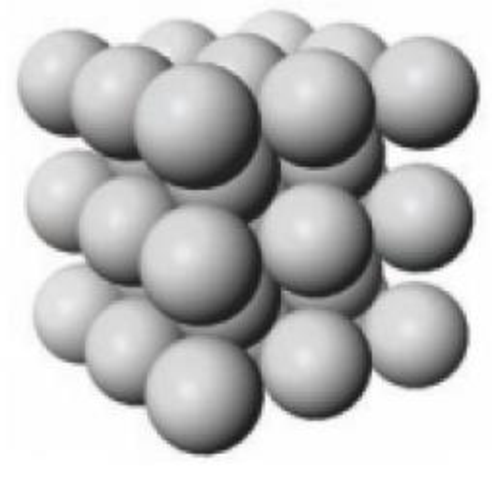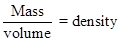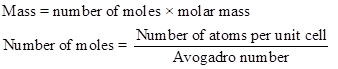Chapter 12, Problem 3PS

Chapter
Section
Textbook Problem

A portion of the crystalline lattice for potassium is illustrated below. (a) In what type of unit cell are the K atoms arranged?A portion of the solid-state structure of potassium. (b) If one edge of the potassium unit cell is 533 pm, what is the density of potassium?

(a)

Interpretation Introduction

Interpretation:

Type of unit cell that are inatoms has to be calculated.

Concept introduction:

Body Centered Cubic Structure:

In simple cubic crystal structure, eight atoms is placed in the corner of the cube and one atom in the center of the cube, therefore it is called as body-centered cubic.

Explanation

The unit cell for tungsten is body- centered cubic. There are atoms at each...

(b)

Interpretation Introduction

Interpretation:

The density of potassium has to be calculated.

Concept introduction:

The volume of the atom is given below,The density of the unit cell is calculated as follows,Still sussing out bartleby?

Check out a sample textbook solution.

See a sample solution

The Solution to Your Study Problems

Bartleby provides explanations to thousands of textbook problems written by our experts, many with advanced degrees!

Get Started